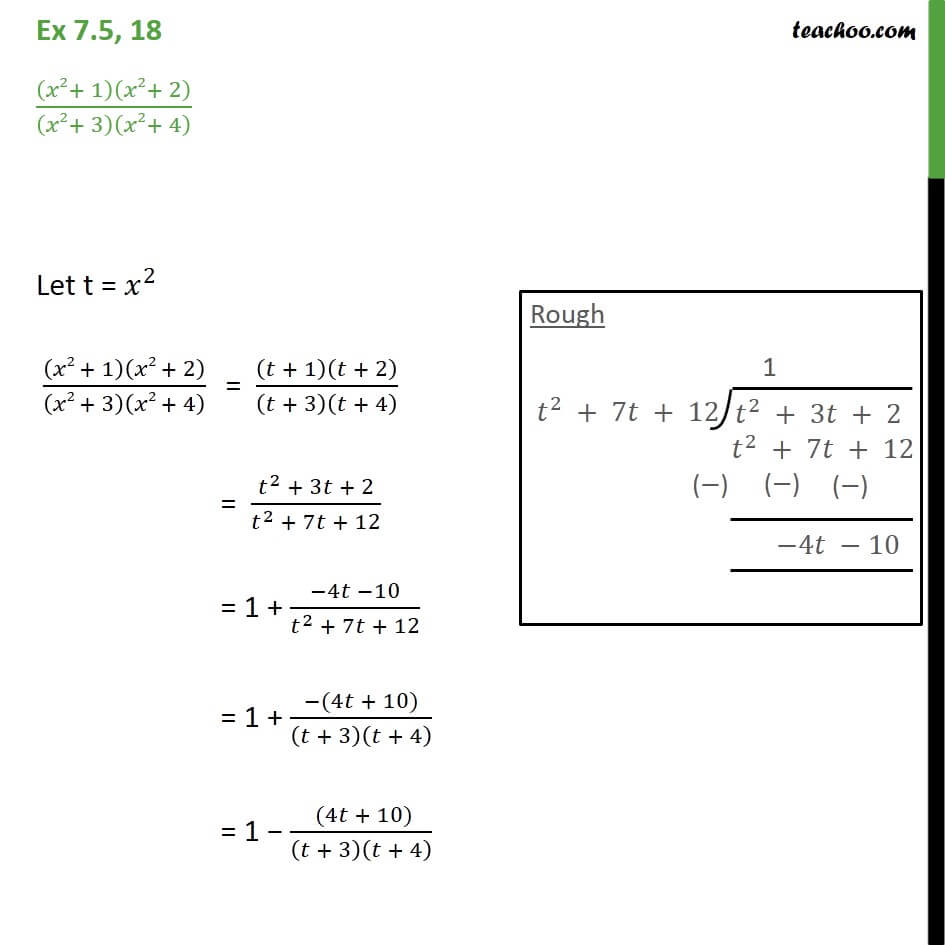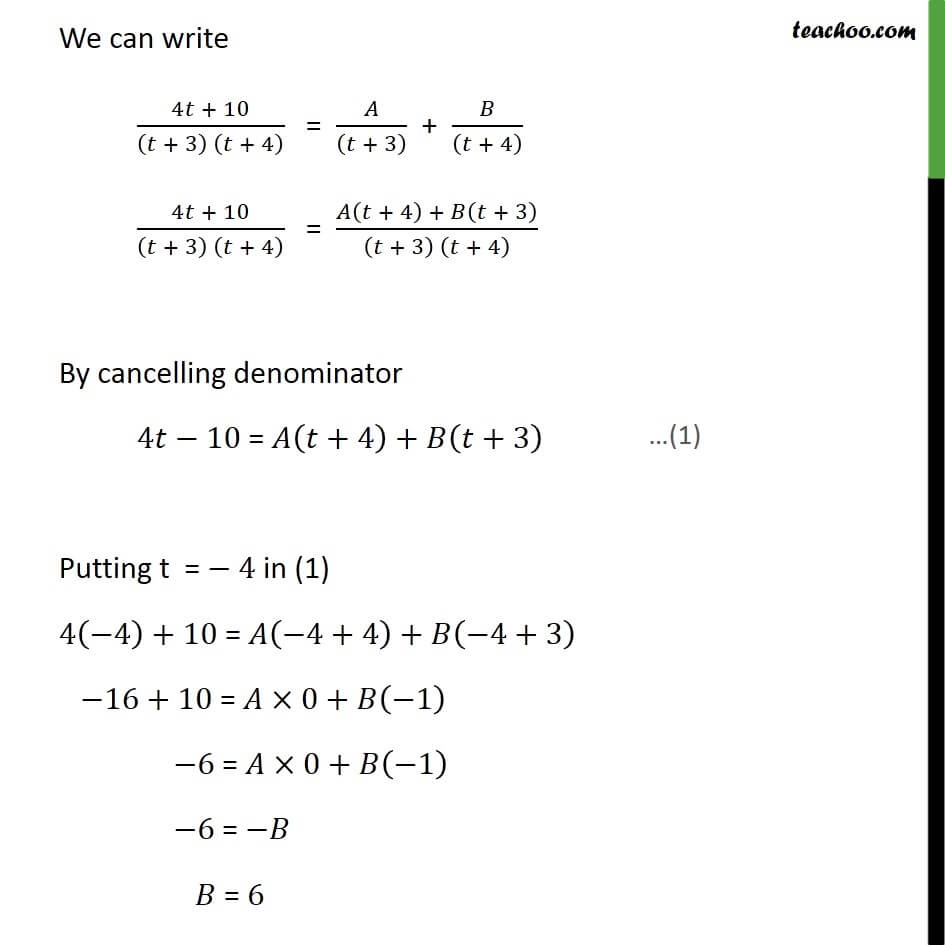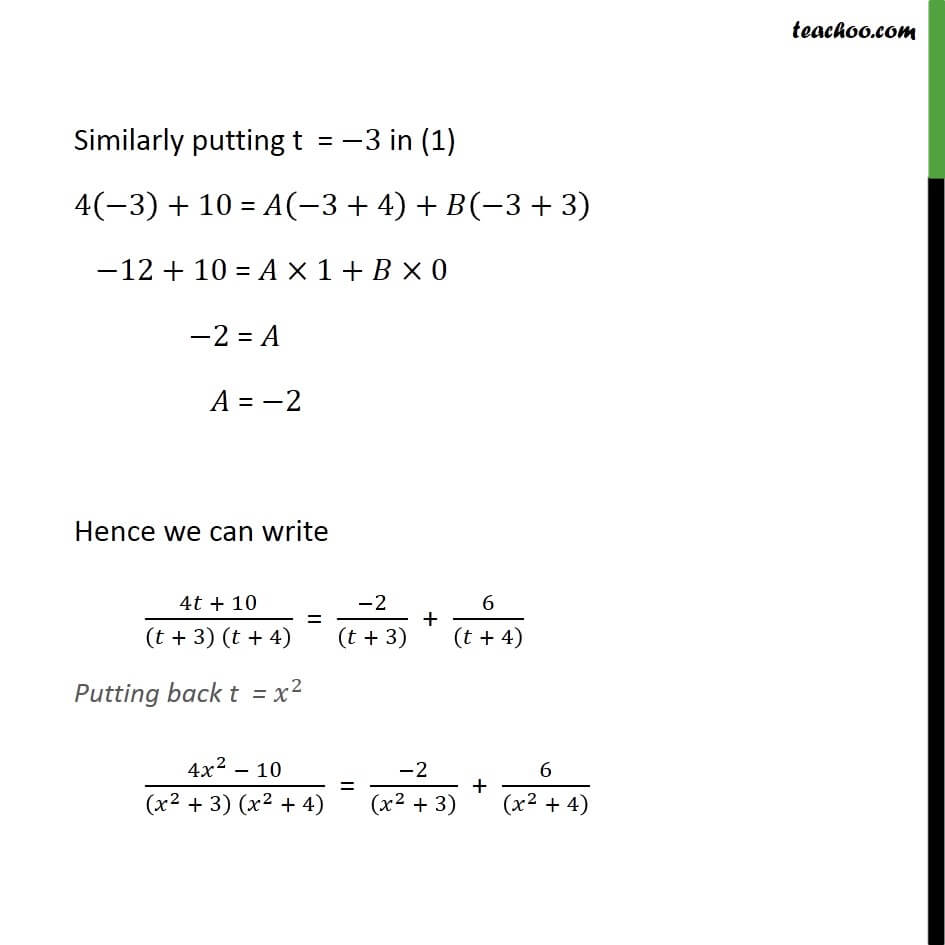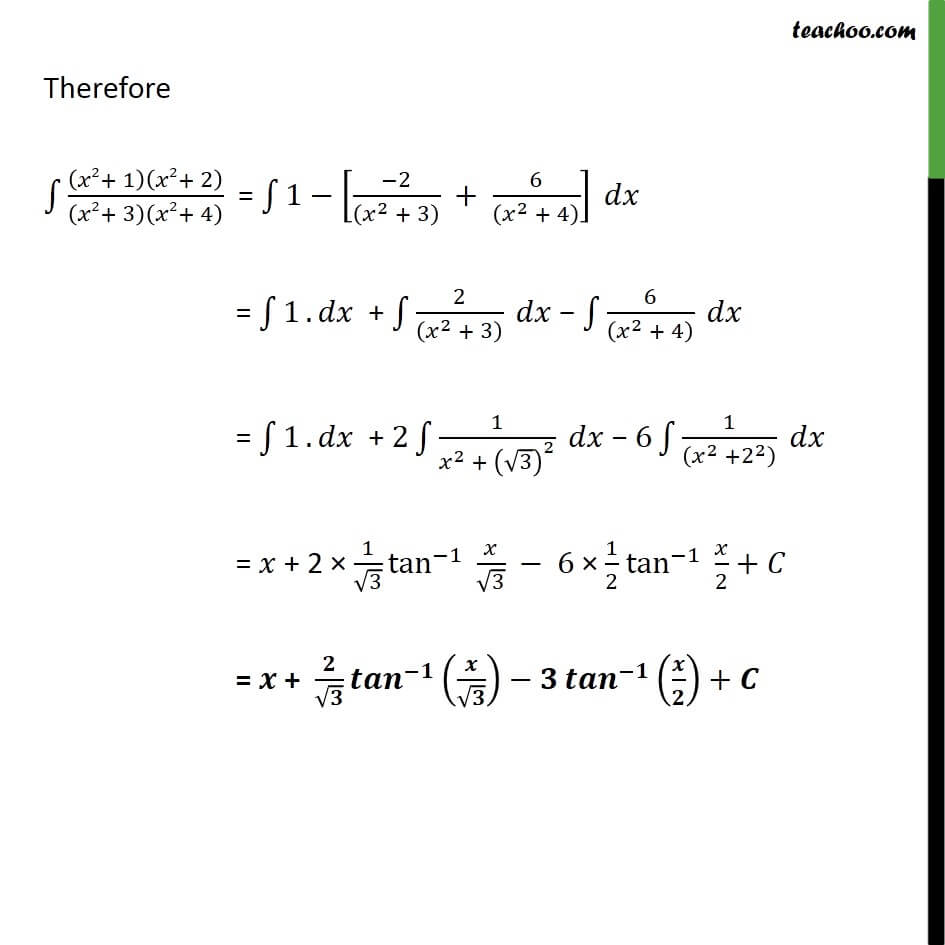1. Chapter 7 Class 12 Integrals
2. Serial order wise
3. Ex 7.5

Transcript

Ex 7.5, 18 2+ 1 2+ 2 2+ 3 2+ 4 Let t = 2 2 + 1 2 + 2 2 + 3 2 + 4 = + 1 + 2 + 3 + 4 = 2 + 3 + 2 2 + 7 + 12 = 1 + 4 10 2 + 7 + 12 = 1 + 4 10 + 3 + 4 We can write 4 + 10 + 3 + 4 = + 3 + + 4 4 + 10 + 3 + 4 = + 4 + + 3 + 3 + 4 By cancelling denominator 4 10 = +4 + +3 Putting t = 4 in (1) 4 4 +10 = 4+4 + 4+3 16+10 = 0+ 1 6 = 0+ 1 6 = = 6 Similarly putting t = 3 in (1) 4 3 +10 = 3+4 + 3+3 12+10 = 1+ 0 2 = = 2 Hence we can write 4 + 10 + 3 + 4 = 2 + 3 + 6 + 4 Putting back t = 2 4 2 10 2 + 3 2 + 4 = 2 2 + 3 + 6 2 + 4 Therefore 2+ 1 2+ 2 2+ 3 2+ 4 = 1 2 2 + 3 + 6 2 + 4 = 1 . + 2 2 + 3 6 2 + 4 = 1 . + 2 1 2 + 3 2 6 1 2 + 2 2 = + 2 1 3 tan 1 3 6 1 2 tan 1 2 + = + +

Ex 7.5

Chapter 7 Class 12 Integrals
Serial order wise